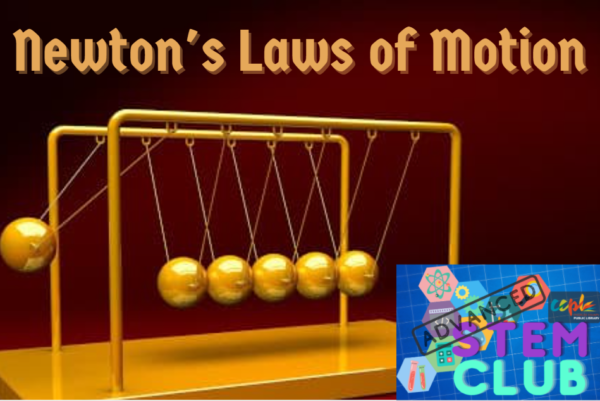Join us on Wednesday, April 28th at 2 p.m. for a fun filled session of Advanced STEM Club, where we will learn even more about astrophysics!

In our next session of Advanced STEM Club, we will be learning all about Sir Isaac Newton’s 3 Laws of Motion and making our own Newton’s Cradles.

### Isaac Newton and Motion

Sir Isaac Newton (1642–1727), the father of the study of dynamics – the study of motion – was one of the greatest scientists and mathematicians that ever lived and is most well-known for his 3 Laws of Motion and his Theory of Gravity. He was born in England on December 25, 1642 or January 4, 1643. He studied and worked in math, physics, and astronomy. His accomplishments in those fields laid the foundations for modern science and revolutionized the world. He died in his sleep at the age of 84 in 1727.

Sir Isaac Newton proposed 3 laws of motion in 1665. These Laws of Motion help to explain how everything in the universe moves.

• If an object is not moving, it will not start moving by itself. If an object is moving, it will not stop or change direction unless something pushes it.

• Objects will move farther and faster when they are pushed harder.

• When an object is pushed in one direction, there is always a resistance of the same size in the opposite direction.

A force is anything that can change the state of motion of an object, like a push or a pull. You use force when you push a letter on the computer keyboard or when you kick a ball. Forces are everywhere. Gravity acts as a constant force on your body, keeping you secure on planet Earth so you don’t float away. To describe a force, we use the direction and strength. For example, when you kick a ball you are exerting force in a specific direction. That is the direction the ball will travel. Also, the harder you kick the ball, the stronger the force you place on it, and the farther it will go.

### First Law of Motion

The first law says that any object in motion will continue to move in the same direction and speed unless forces act on it.  That means if you kick a ball, it will fly forever unless some sort of forces act on it! As strange as this may sound, it’s true. When you kick a ball, forces start to act on it immediately. These include resistance or friction from the air and gravity. Gravity pulls the ball down to the ground and the air resistance slows it down.

### Second Law of Motion

The second law states that the greater the mass of an object, the more force it will take to accelerate the object. There is even an equation that says Force = mass x acceleration or F=ma.  This also means that the harder you kick a ball the farther it will go. This seems kind of obvious to us, but having an equation to figure out the math and science is very helpful to scientists

### Third Law of Motion

The third law states that for every action, there is an equal and opposite reaction. This means that there are always two forces that are the same. In the example where you kicked the ball, there is the force of your foot on the ball, but there is also the same amount of force that the ball puts on your foot. This force is in the exact opposite direction.

### Today we will be making our own Newton’s Cradle

How does a Newton’s Cradle work? The device can be explained with some of the fundamental principles of physics and mechanics (as theorized by Sir Isaac Newton, René Descartes and others).

Newton’s Cradle aptly demonstrates the principle of the conservation of momentum (mass times speed). This principle states that when two objects collide, the total momentum of the objects before the collision is equal to the total momentum of the objects after the collision.

In other words, when the first ball of Newton’s Cradle collides with the second, the first ball stops, but its momentum isn’t lost, just transferred to the second ball, then the third, then the fourth, until it reaches the very last ball. You witness this conservation of momentum as the last ball swings into the air with nearly the same momentum as the first ball.  Thus, if two balls are lifted into the air on one end of the device and released, then two balls on the opposite end will swing in response.

This continuous clicking of balls is also proof of Newton’s law of the conservation of energy, which states that energy can’t be created or destroyed but that it can change forms. Newton’s Cradle demonstrates this last part of the law quite well, as it converts the potential energy of one ball into kinetic energy that is transferred down the line of balls and ultimately results in the upward swinging of the last ball.

• 5 marbles (or some kind of small ball)

• 12-15 popsicle/craft sticks

• 1 plastic or paper straw

• Yarn or string

• Scissors

• Hot glue gun (if you do not have a hot glue gun you can use craft glue but it will take much longer for your parts to dry to be able to assemble your Newton’s Cradle)